# SAT Math Multiple Choice Question 954: Answer and Explanation

### Test Information

Question: 954

6.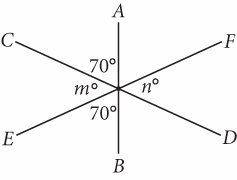In the figure above,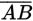,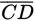, and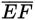all intersect. What is the value of n?

• A. 30
• B. 40
• C. 50
• D. 60

Getting to the Answer: The three angles on the left sideform a straight line, so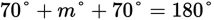. Solving for m gives 40. The angles marked m° and n° are vertical angles, so n = 40 as well. (B) is correct.